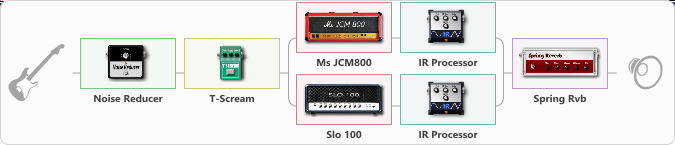# my preset

Discussion in 'ToneLib-GFX presets' started by THE FREEMAN MUSIC TFG, Apr 12, 2020.

1. ### THE FREEMAN MUSIC TFGNew Member

my preset

Preset name: Empty Preset

Effects chain:Effect: "Noise Reducer" (Dynamics / Filter), active - "yes"
{
"Sens" = 100
"Mode" = Hard
}

Effect: "T-Scream" (Overdrive / Distortion), active - "yes"
{
"Drive" = 0
"Tone" = 65
"Level" = 100
}

Effect: "Splitter" (Dynamics / Filter), active - "yes"
{
"A-Bypass" = Off
"A-Pan" = 0
"A-Level" = 55
"B-Bypass" = Off
"B-Pan" = 0
"B-Level" = 55

'A' branch:
{

Effect: "Ms JCM800" (Amp simulators), active - "yes"
{
"Gain" = 50
"Bass" = 50
"Middle" = 50
"Treble" = 50
"Presence" = 50
"Master" = 50
"Level (dB)" = 0
}

Effect: "IR Processor" (Cabinets), active - "yes"
{
"IR" = Плотный 1
"Low Cut (Hz)" = 0
"Hi Cut (kHz)" = 20.0
"Mix" = 100
"Level (dB)" = 0
}
}
'B' branch:
{

Effect: "Slo 100" (Amp simulators), active - "yes"
{
"Gain" = 42
"Bass" = 39
"Middle" = 58
"Treble" = 70
"Presence" = 50
"Master" = 57
"Level (dB)" = 8
}

Effect: "IR Processor" (Cabinets), active - "yes"
{
"IR" = Сбалансированный
"Low Cut (Hz)" = 0
"Hi Cut (kHz)" = 20.0
"Mix" = 100
"Level (dB)" = 0
}
}
}

Effect: "Spring Rvb" (Reverb), active - "yes"
{
"Time" = 5.7
"PreDelay" = 0
"LoDamp" = 16
"HiDamp" = 26
"Mix" = 20
}

Note: You will need to download and install the ToneLib-GFX software to use the preset.

File size:
20.8 KB
Views:
3,388